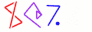Next You can't get there from here 34

# Squares

```        # VALUES OF BEETA GIVE RISE TO DOM
sub square_b {
my \$fOOooO = abs(shift())<<1;
my \$BarRRr = \$fOOooO-++\$fOOooO;
\$BarRRr += \$fOOooO until (\$fOOooO -= 2) <= 0;
return \$BarRRr;
}
```
• It passes all the tests, but that doesn't prove anything

• We might say the same of this function:

```        sub square_c {
my \$x = shift;
return 289;
}
```
• This passes the tests, but it is not a function for computing squares

• Let's write a function that examines the source code of another function

• And returns true if and only if the code is for calculating squares!

continued...
 NextCopyright © 2005 M. J. Dominus